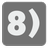Friendly Number
Elementary+
English FR RULong numbers can be made to look nicer, so let’s write some code to do just that.

You should write a function for converting a number to string using several rules. First of all, you will need to cut the number with a given base ( base argument; default 1000). The value is a float number with decimal after the point ( decimals argument; default 0). For the value, use the rounding towards zero rule (5.6⇒5, -5.6⇒-5) if the decimal = 0, otherwise use the standard rounding procedure. If the number of decimals is greater than the current number of digits after dot, trail value with zeroes. The number should be a value with letters designating the power. You will be given a list of power designations ( powers argument; default ['', 'k', 'M', 'G', 'T', 'P', 'E', 'Z', 'Y']). If you are given suffix ( suffix argument; default ‘’) , then you must append it. If you don’t have enough powers - stay at the maximum. And zero is always zero without powers, but with suffix.

Let's look at examples. It will be simpler.

• n=102
result: "102", the base is default 1000 and 102 is lower this base.
• n=10240
result: "10k", the base is default 1000 and rounding down.
• n=12341234, decimals=1
result: "12.3M", one digit after the dot.
• n=12000000, decimals=3
result: "12.000M", trailing zeros.
• n=12461, decimals=1
result: "12.5k", standard rounding.
• n=1024000000, base=1024, suffix='iB'
result: '976MiB', the different base and the suffix.
• n=-150, base=100, powers=['', 'd', 'D']
result: '-1d', the negative number and rounding towards zero.
• n=-155, base=100, decimals=1, powers=['', 'd', 'D']
result: '-1.6d', the negative number and standard rounding.
• n=255000000000, powers=['', 'k', 'M']
result: '255000M', there is not enough powers.

Input: A number as an integer. The keyword argument "base" as an integer, default 1000. The keyword argument "decimals" as an integer, default 0. The keyword argument "suffix" as a string, default ''. The keyword argument "powers" as a list of string, default ['', 'k', 'M', 'G', 'T', 'P', 'E', 'Z', 'Y'].

Output: The converted number as a string.

Example:

```friendly_number(102) == '102'
friendly_number(10240) == '10k'
friendly_number(12341234, decimals=1) == '12.3M'
friendly_number(12000000, decimals=3) == '12.000M'
friendly_number(12461,...```
You should be an authorized user in order to see the full description and start solving this mission.

## Become Awesome

• No Limits
• More Content10
Settings
Code:
Other:
CheckiO Extensions

CheckiO Extensions allow you to use local files to solve missions. More info in a blog post.

In order to install CheckiO client you'll need installed Python (version at least 3.8)

Install CheckiO Client first:

`pip3 install checkio_client`

`checkio --domain=py config --key=`

Sync solutions into your local folder

`checkio sync`

(in beta testing) Launch local server so your browser can use it and sync solution between local file end extension on the fly. (doesn't work for safari)

`checkio serv -d`

Alternatevly, you can install Chrome extension or FF addon

`checkio install-plugin`
`checkio install-plugin --ff`
`checkio install-plugin --chromium`

Read more here about other functionality that the checkio client provides. Feel free to submit an issue in case of any difficulties.